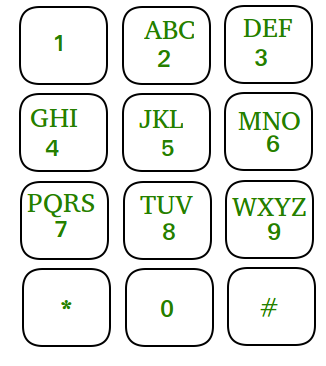# Convert a sentence into its equivalent mobile numeric keypad sequence

Given a sentence in the form of a string, convert it into its equivalent mobile numeric keypad sequence.Examples :

```Input : GEEKSFORGEEKS
Output : 4333355777733366677743333557777
For obtaining a number, we need to press a
number corresponding to that character for
number of times equal to position of the
character. For example, for character C,
we press number 2 three times and accordingly.

Input : HELLO WORLD
Output : 4433555555666096667775553
```

## Recommended: Please try your approach on {IDE} first, before moving on to the solution.

Follow the steps given below to convert a sentence into its equivalent mobile numeric keypad sequence.

• For each character, store the sequence which should be obtained at its respective position in an array, i.e. for Z, store 9999. For Y, store 999. For K, store 55 and so on.
• For each character, subtract ASCII value of ‘A’ and obtain the position in the array pointed
by that character and add the sequence stored in that array to a string.
• If the character is a space, store 0
• Print the overall sequence.

Below is the implementation of above method :

## C++

 `// C++ implementation to convert a ` `// sentence into its equivalent ` `// mobile numeric keypad sequence ` `#include ` `using` `namespace` `std; ` ` `  `// Function which computes the sequence ` `string printSequence(string arr[], ` `                       ``string input) ` `{ ` `    ``string output = ``""``; ` ` `  `    ``// length of input string ` `    ``int` `n = input.length(); ` `    ``for` `(``int` `i=0; i

## Java

 `// Java implementation to convert a ` `// sentence into its equivalent ` `// mobile numeric keypad sequence ` `import` `java.util.*; ` ` `  `class` `GFG ` `{ ` ` `  `    ``// Function which computes the sequence ` `    ``static` `String printSequence(String arr[], ` `                               ``String input) ` `    ``{ ` `        ``String output = ``""``; ` `     `  `        ``// length of input string ` `        ``int` `n = input.length(); ` `        ``for` `(``int` `i = ``0``; i < n; i++) ` `        ``{ ` `            ``// Checking for space ` `            ``if` `(input.charAt(i) == ``' '``) ` `                ``output = output + ``"0"``; ` `     `  `            ``else` `            ``{ ` `                ``// Calculating index for each  ` `                ``// character ` `                ``int` `position = input.charAt(i) - ``'A'``; ` `                ``output = output + arr[position]; ` `            ``} ` `        ``} ` `     `  `        ``// Output sequence ` `        ``return` `output; ` `    ``} ` `     `  `    ``// Driver Function ` `    ``public` `static` `void` `main(String[] args) ` `    ``{ ` `        ``// storing the sequence in array ` `        ``String str[] = {``"2"``,``"22"``,``"222"``, ` `                        ``"3"``,``"33"``,``"333"``, ` `                        ``"4"``,``"44"``,``"444"``, ` `                        ``"5"``,``"55"``,``"555"``, ` `                        ``"6"``,``"66"``,``"666"``, ` `                        ``"7"``,``"77"``,``"777"``,``"7777"``, ` `                        ``"8"``,``"88"``,``"888"``, ` `                        ``"9"``,``"99"``,``"999"``,``"9999"` `                    ``}; ` ` `  `        ``String input = ``"GEEKSFORGEEKS"``; ` `        ``System.out.println(printSequence(str, input)); ` `    ``} ` `} ` ` `  `// This code is contributed by Gitanjali. `

## Python3

 `# Python3 implementation to convert ` `# a sentence into its equivalent ` `# mobile numeric keypad sequence ` ` `  `# Function which computes the  ` `# sequence ` `def` `printSequence(arr, ``input``): ` ` `  `# length of input string ` `    ``n ``=` `len``(``input``) ` `    ``output ``=` `"" ` `     `  `    ``for` `i ``in` `range``(n): ` `     `  `        ``# checking for space ` `        ``if``(``input``[i] ``=``=` `' '``): ` `            ``output ``=` `output ``+` `"0"` `        ``else``: ` `            ``# calculating index for each ` `            ``# character          ` `            ``position ``=` `ord``(``input``[i]) ``-` `ord``(``'A'``) ` `            ``output ``=` `output ``+` `arr[position] ` `    ``# output sequence      ` `    ``return` `output ` `     `  `# Driver code ` `str` `=` `[``"2"``, ``"22"``, ``"222"``, ` `       ``"3"``, ``"33"``, ``"333"``, ` `       ``"4"``, ``"44"``, ``"444"``, ` `       ``"5"``, ``"55"``, ``"555"``, ` `       ``"6"``, ``"66"``, ``"666"``, ` `       ``"7"``, ``"77"``, ``"777"``, ``"7777"``, ` `       ``"8"``, ``"88"``, ``"888"``, ` `       ``"9"``, ``"99"``, ``"999"``, ``"9999"` `] ` ` `  `input` `=` `"GEEKSFORGEEKS"``; ` `print``( printSequence(``str``, ``input``)) ` ` `  `# This code is contributed by upendra bartwal `

## C#

 `// C# implementation to convert a ` `// sentence into its equivalent ` `// mobile numeric keypad sequence ` `using` `System; ` ` `  `class` `GFG ` `{ ` ` `  `    ``// Function which computes the sequence ` `    ``static` `String printSequence(``string` `[]arr, ` `                            ``string` `input) ` `    ``{ ` `        ``string` `output = ``""``; ` `     `  `        ``// length of input string ` `        ``int` `n = input.Length; ` `        ``for` `(``int` `i = 0; i < n; i++) ` `        ``{ ` `            ``// Checking for space ` `            ``if` `(input[i] == ``' '``) ` `                ``output = output + ``"0"``; ` `     `  `            ``else` `            ``{ ` `                ``// Calculating index for each  ` `                ``// character ` `                ``int` `position = input[i] - ``'A'``; ` `                ``output = output + arr[position]; ` `            ``} ` `        ``} ` `     `  `        ``// Output sequence ` `        ``return` `output; ` `    ``} ` `     `  `    ``// Driver Function ` `    ``public` `static` `void` `Main() ` `    ``{ ` `        ``// storing the sequence in array ` `        ``string` `[]str = {``"2"``,``"22"``,``"222"``, ` `                        ``"3"``,``"33"``,``"333"``, ` `                        ``"4"``,``"44"``,``"444"``, ` `                        ``"5"``,``"55"``,``"555"``, ` `                        ``"6"``,``"66"``,``"666"``, ` `                        ``"7"``,``"77"``,``"777"``,``"7777"``, ` `                        ``"8"``,``"88"``,``"888"``, ` `                        ``"9"``,``"99"``,``"999"``,``"9999"` `                    ``}; ` ` `  `        ``string` `input = ``"GEEKSFORGEEKS"``; ` `        ``Console.WriteLine(printSequence(str, input)); ` `    ``} ` `} ` ` `  `// This code is contributed by vt_m. `

## PHP

 ` `

Output :

```4333355777733366677743333557777
```

Time complexity : O(n)

Attention reader! Don’t stop learning now. Get hold of all the important DSA concepts with the DSA Self Paced Course at a student-friendly price and become industry ready.

My Personal Notes arrow_drop_upCheck out this Author's contributed articles.

If you like GeeksforGeeks and would like to contribute, you can also write an article using contribute.geeksforgeeks.org or mail your article to contribute@geeksforgeeks.org. See your article appearing on the GeeksforGeeks main page and help other Geeks.

Please Improve this article if you find anything incorrect by clicking on the "Improve Article" button below.

Improved By : chitranayal

Article Tags :
Practice Tags :

2

Please write to us at contribute@geeksforgeeks.org to report any issue with the above content.